NEET  >  NCERT Based Test: Motion in Combined Electric & Magnetic Fields

# NCERT Based Test: Motion in Combined Electric & Magnetic Fields

Test Description

## 15 Questions MCQ Test Physics Class 12 | NCERT Based Test: Motion in Combined Electric & Magnetic Fields

NCERT Based Test: Motion in Combined Electric & Magnetic Fields for NEET 2023 is part of Physics Class 12 preparation. The NCERT Based Test: Motion in Combined Electric & Magnetic Fields questions and answers have been prepared according to the NEET exam syllabus.The NCERT Based Test: Motion in Combined Electric & Magnetic Fields MCQs are made for NEET 2023 Exam. Find important definitions, questions, notes, meanings, examples, exercises, MCQs and online tests for NCERT Based Test: Motion in Combined Electric & Magnetic Fields below.
Solutions of NCERT Based Test: Motion in Combined Electric & Magnetic Fields questions in English are available as part of our Physics Class 12 for NEET & NCERT Based Test: Motion in Combined Electric & Magnetic Fields solutions in Hindi for Physics Class 12 course. Download more important topics, notes, lectures and mock test series for NEET Exam by signing up for free. Attempt NCERT Based Test: Motion in Combined Electric & Magnetic Fields | 15 questions in 15 minutes | Mock test for NEET preparation | Free important questions MCQ to study Physics Class 12 for NEET Exam | Download free PDF with solutions
 1 Crore+ students have signed up on EduRev. Have you?
NCERT Based Test: Motion in Combined Electric & Magnetic Fields - Question 1

### A charged particle with charge q enters a region of constant, uniform and mutually orthogonal fields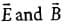with a velocity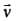perpendicular to both, and comes out without any change in its magnitude or direction. Then

Detailed Solution for NCERT Based Test: Motion in Combined Electric & Magnetic Fields - Question 1

When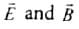are perpendicular and velocity has no change then qE = qvB i.e. v= E/B. The two forces oppose each other so, v is along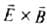i.e.,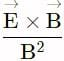.
As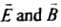are perpendicular to each other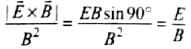NCERT Based Test: Motion in Combined Electric & Magnetic Fields - Question 2

### A charged particle would continue to move with a constant velocity in a region wherein, which of the following conditions is not correct?

NCERT Based Test: Motion in Combined Electric & Magnetic Fields - Question 3

### The cyclotron frequency vc is given by

Detailed Solution for NCERT Based Test: Motion in Combined Electric & Magnetic Fields - Question 3

In cyclotron the centripetal force is balanced by magnetic force, then
qvB = mn2/r ⇒ qBr/m = v
where v = 2πrv∴ vc = qB/2πm

NCERT Based Test: Motion in Combined Electric & Magnetic Fields - Question 4

A charged particle is moving in a cyclotron, what effect on the radius of path of this charged particle will occur when the frequency of the radio frequency field is doubled?

Detailed Solution for NCERT Based Test: Motion in Combined Electric & Magnetic Fields - Question 4

As frequency of revolution in a cyclotron
vC = Bq/2πm is independent of r.
So the radius of path in the dees will remain unchanged. When the frequency is changed.

NCERT Based Test: Motion in Combined Electric & Magnetic Fields - Question 5

A cubical region of space is filled with some uniform electric and magnetic field. An electron enters the cube across one of its faces with velocity v and a positron enters via opposite face with velocity - v. At this instant, which one of the following is not correct?

NCERT Based Test: Motion in Combined Electric & Magnetic Fields - Question 6

A cyclotron is operated at an oscillator frequency of 12 MHz and has a dee radius R = 50 cm. What is the magnitude of the magnetic field needed for a proton to be accelerated in the cyclotron?

NCERT Based Test: Motion in Combined Electric & Magnetic Fields - Question 7

If an electron is moving in a magnetic field of 5.4 x 10-4 T on a circular path of radius 32 cm having a frequency of 2.5 MHz, then its speed will be

Detailed Solution for NCERT Based Test: Motion in Combined Electric & Magnetic Fields - Question 7

Here, B = 5.4 x 10-4 T;
r = 32 cm = 32 x 10-2 m; v = 2.5 MHz = 2.5 x 106Hz
The speed of electron on circular path
v = r x 2πv = 32 x 10-2 x 2 x 3.14 x 2.5 x 106
= 502.4 x 104 = 5.024 x 106 m s-1

NCERT Based Test: Motion in Combined Electric & Magnetic Fields - Question 8

A proton and an α-partide enter in a unifoi magnetic field perpendicularly with same speed. The ratio of time periods of both particle (TP/Tα) will be

Detailed Solution for NCERT Based Test: Motion in Combined Electric & Magnetic Fields - Question 8

The time period of revolution of a charged particle in a magnetic field is
T = 2πm/Bq
For proton, mP = m, qP = q ; ∴ TP = 2πm/Bq
Now, for α-particle, mα = 4m, qα = 2q
∴ Tα = (2π(4m))/(B(Bq)) = 2(2πm/Bq) ⇒ TP/Tα = 1/2

NCERT Based Test: Motion in Combined Electric & Magnetic Fields - Question 9

A proton, a deutron and an α-particle with same, kinetic energy enter perpendicularly in a uniform magnetic field, then the ratio of radii of their circular paths is

Detailed Solution for NCERT Based Test: Motion in Combined Electric & Magnetic Fields - Question 9

∵ r =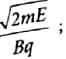∴ r ∝ √m/q
Hence, rp : rd ; rα =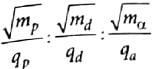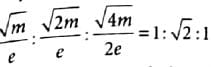NCERT Based Test: Motion in Combined Electric & Magnetic Fields - Question 10

If an electron enters with a velocity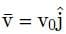into a cubical region in a uniform electromagnetic field and the orbit of the electron is found to spiral down inside the cube in plane parallel to x-y plane then the configuration of electric field and magnetic field is

Detailed Solution for NCERT Based Test: Motion in Combined Electric & Magnetic Fields - Question 10

The orbit of the electron spirals down inside the cube in the plane parallel to x−y plane. Therefore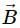must be along +z  direction and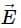should be along x−axis.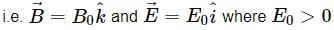NCERT Based Test: Motion in Combined Electric & Magnetic Fields - Question 11

The energy of emergent protons in MeV from a cyclotron having radius of its dees 1.8 m and applied magnetic field 0.7T is (mass of proton = 1.67×10−27 kg):

Detailed Solution for NCERT Based Test: Motion in Combined Electric & Magnetic Fields - Question 11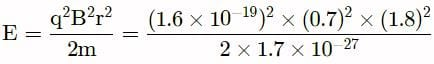= 1.22 x 10-11 j = 76 MeV

NCERT Based Test: Motion in Combined Electric & Magnetic Fields - Question 12

Which of the following is not correct about cyclotron?

Detailed Solution for NCERT Based Test: Motion in Combined Electric & Magnetic Fields - Question 12

The charged particles and ions in cyclotron can move along circular path only.

NCERT Based Test: Motion in Combined Electric & Magnetic Fields - Question 13

The operating magnetic field for accelerating protons in a cyclotron oscillator having frequency of 12 MHz is (q = 1.6 x 10-19C, mp = 1.67 x 10-27 kg and 1 MeV = 1.6 x 10-13 j)

Detailed Solution for NCERT Based Test: Motion in Combined Electric & Magnetic Fields - Question 13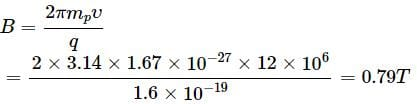NCERT Based Test: Motion in Combined Electric & Magnetic Fields - Question 14

In the question number 34, the kinetic energy (in MeV) of the proton beam produced by the accelerator is (radius of dees = 60 cm)

Detailed Solution for NCERT Based Test: Motion in Combined Electric & Magnetic Fields - Question 14

Final velocity of proton is
v = r x 2πv = 0.6 x 2 x 3.14 x 12 x 106 = 4.5 x 107 m s-1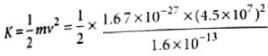= 10.6 MeV

NCERT Based Test: Motion in Combined Electric & Magnetic Fields - Question 15

An electron is moving in a cyclotron at a speed of 3.2 x 107 m s-1 in a magnetic field of 5 x 10-4 T perpendicular to it. What is the frequency of this electron?
(q = 1.6 x 10-19 C, me = 9.1 x 10-31 kg)

Detailed Solution for NCERT Based Test: Motion in Combined Electric & Magnetic Fields - Question 15

v = 3.2 x 107 m s-1 ;B = 5 x 10-4 T
The frequency of electron is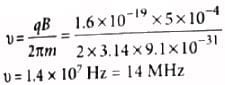## Physics Class 12

157 videos|452 docs|213 tests
 Use Code STAYHOME200 and get INR 200 additional OFF Use Coupon Code
Information about NCERT Based Test: Motion in Combined Electric & Magnetic Fields Page
In this test you can find the Exam questions for NCERT Based Test: Motion in Combined Electric & Magnetic Fields solved & explained in the simplest way possible. Besides giving Questions and answers for NCERT Based Test: Motion in Combined Electric & Magnetic Fields, EduRev gives you an ample number of Online tests for practice

## Physics Class 12

157 videos|452 docs|213 tests

### How to Prepare for NEET

Read our guide to prepare for NEET which is created by Toppers & the best Teachers